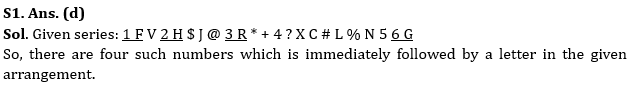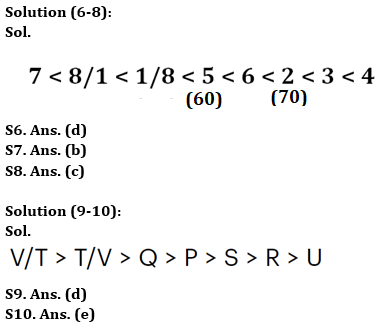Latest Banking jobs   »

# Reasoning Quizzes Quiz For IRDA AM 2023-23rd May

Directions (1-5): Study the following arrangement carefully and answer the questions accordingly.

1 F V 2 H \$ J @ 3 R * + 4 ? X C # L % N 5 6 G

Q1. How many such numbers are immediately followed by a letter in the given arrangement?
(a) One
(b) Two
(c) Three
(d) Four
(e) None of the above

Q2. If all the symbols are dropped, then which of the following will be 8th element to the left of 7th element from right end in the given arrangement?
(a) 3
(b) F
(c) V
(d) 1
(e) None of the above

Q3. In the given arrangement, how many letters are immediately followed & preceded by a number?
(a) One
(b) Two
(c) Three
(d) Four
(e) None of the above

Q4. What will be the sum of all even numbers in the given arrangement?
(a) 10
(b) 14
(c) 12
(d) 8
(e) None of the above

Q5. How many such symbols are immediately followed by a consonant and immediately preceded by a vowel in the given arrangement?
(a) One
(b) Two
(c) Three
(d) Four
(e) None of the above

Directions (6-8): Answer the questions based on the information given below.
There are 8 storages (1, 2, 3, 4, 5, 6, 7 and 8) of Rice are arranged in descending order of their weight from right to left. 5 is lighter than 6 but heavier than 8 and 1. 2 is heavier than 6. 7 is the lightest. 3 is heavier than 2 but not the heaviest. 4 is heavier than 6. The third heaviest weighs 70kg. There is a difference of 10kg between 5 and 2.

Q6. How many storages are heavier than 5?
(a) One
(b) Two
(c) Three
(d) Four
(e) None of the above

Q7. What is the possible weight of storage 6?
(a) 59kg
(b) 65kg
(c) 71kg
(d) 75kg
(e) None of the above

Q8. Which among the following is 2nd heaviest?
(a) 2
(b) 6
(c) 3
(d) 4
(e) None of the above

Directions (9-10): Answer the questions based on the information given below.
Seven persons P, Q, R, S, T, U, and V have different heights. Equal number of persons is taller and shorter than P. S is taller than R and U. Only V and T are taller than Q. R is not the shortest.

Q9. How many persons are taller than S?
(a) One
(b) Two
(c) Three
(d) Four
(e) None of the above

Q10. Who among the following is tallest?
(a) S
(b) R
(c) V
(d) T
(e) Cannot be determined

SolutionsS2. Ans. (b)
Sol. New arrangement: 1 F V 2 H J 3 R 4 X C L N 5 6 G
So, 8th element to the left of 7th element from right end in the given arrangement is F.

S3. Ans. (e)
Sol. There is no such letter which is immediately followed & preceded by a number.

S4. Ans. (c)
Sol. Given series: 1 F V 2 H \$ J @ 3 R * + 4 ? X C # L % N 5 6 G
Sum of even numbers = (2 + 4 + 6) = 12.

S5. Ans. (e)
Sol. There is no symbol which is immediately followed by a consonant and immediately preceded by a vowel in the given arrangement.## FAQs

### How many sections are there in the IRDAI exam?

There are 4 sections in the IRDAI assistant manager online preliminary exam. They are Reasoning, English Language, General awareness, Quantitative aptitude.

#### Congratulations!Union Budget 2023-24: Free PDF